# 3 Cosmological Field Equations

In the theory of general relativity, we describe the local nature of gravitational field nearby matter as an algebraic relation between the Ricci curvature and the matter fields, i.e., the Einstein field equations:(26)

where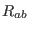is the Ricci curvature,the energy-momentum of the matter fields, andthe trace of the energy-momentum tensor.

The successive contractions of Eq. (26) on using of Eq. (24) lead to a set of relations:(27)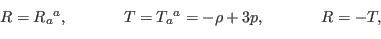(28)

where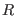is the Ricci scalar. The Ricci curvature is derived from the once contracted Riemann curvature tensor: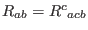.

The Riemann tensor is split into symmetric (massless) traceless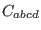and traceful massive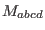parts: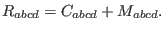(29)

The symmetric traceless part of the Riemann curvature is called the Weyl conformal curvature with the following properties: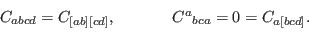(30)

The nonlocal (long-range) fields, the parts of the curvature not directly determined locally by matter, are given by the Weyl curvature; propagating the Newtonian (and anti-Newtonian) forces and gravitational waves. It can be shown that the Weyl tensoris irreducibly split into the Newtonian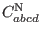and the anti-Newtonian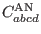parts: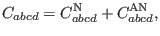(31)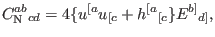(32)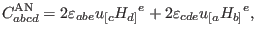(33)

where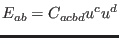is the gravitoelectric field and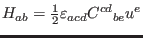the gravitomagnetic field. They are spacelike and traceless symmetric.

The traceful massive part of the Riemann curvature consists of the matter fields and the characteristics of local interactions with matter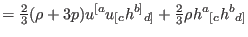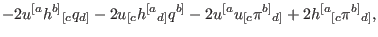(34)

Therefore, the Weyl curvature is linked to the matter fields through the Riemann curvature.

Subsections
Ashkbiz Danehkar
2018-03-26